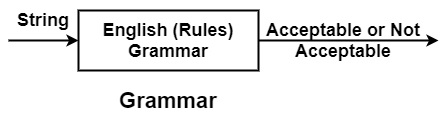# What is Context-Free Grammar?

Grammar − It is a set of rules which checks whether a string belongs to a particular language a not.

A program consists of various strings of characters. But, every string is not a proper or meaningful string. So, to identify valid strings in a language, some rules should be specified to check whether the string is valid or not. These rules are nothing but make Grammar.

Example − In English Language, Grammar checks whether the string of characters is acceptable or not, i.e., checks whether nouns, verbs, adverbs, etc. are in the proper sequence.## Context-Free Grammar

It is a notation used to specify the syntax of the language. Context-free Grammar is used to design parsers.

As Lexical Analyzer generates a string of tokens which are given to parser to construct parse tree. But, before constructing the parse tree, these tokens will be grouped so that the results of grouping will be a valid construct of a language. So, to specify constructs of language, a suitable notation is used, which will be precise & easy to understand. This notation is Context-Free Grammar.

Formally, Context-Free Grammar (G) can be defined as −

It is a 4-tuple (V,∑,P,S)

• V is a set of Non-Terminals or Variables
• ∑ is a set of terminals.
• P is a set of Productions or set of rules
• S is a starting symbol

G is context Free if every Production (P) is of form A → α, where A∈V and α ∈(V∪ ∑ )*.

Example1 − Write down Grammar for language

L={an|n≥1}

Solution

Let G=(V,Σ,P,S)
V = {S}
Σ={a}
P = {
S→aS
S→a
}

These productions generate language an.

i.e., S ⇒ a
S ⇒ a S ⇒ a a or a2
S ⇒ a S ⇒ a a S ⇒ a a a or a3
.
.
.
S ⇒ a S ⇒ a a S ⇒ a a a S ⇒ ... ⇒ an

In Context-Free Grammar, variable or Non-Terminal symbols occur at the left side of →(Arrow). These symbols will be expanded until all terminal symbols will be generated.

The terminal symbols are tokens used in a language.

Example2 − Find out language generated by Grammar.

G=({S},{a,b}{S → a S b,S → a,b},S)

Solution

S ⇒ a $\underline{S}$ b

⇒ a $\underline{aSb}$ b

⇒ a a a $\underline{S}$ b b b

⇒ a a a a S b b b b

………………………

⇒ an−1$\underline{S}$ bn−1

⇒ an−1 $\underline{a\:b}$ bn−1

⇒ an bn

∴ Language L= {an bn| 𝑛≥2}

Example3 − Write down CFG G generating all integers.

Solution

G=(V,∑,P,S)

V = {S, <sign>, <digit>, <integer>}

Σ = {0, 1, 2, 3,……………………..9, +, - }

$P= \begin{Bmatrix} \:\:\:\:S → <sign> <integer>\ \:\:\:\:\:\:\:\:<sign>→ +|−\ <integer> → <digit> <integer>|<digit>\ \:\:\:\: <digit> → 0 | 1 | 2 | 3…………|9 \end{Bmatrix}$

For example, the derivation for – 12 is given by −

S ⇒ <sign> <integer>
⟹ −<integer>
⟹ −<digit> <integer>
⟹ −1<integer>
⟹ −1<digit>⟹−12.